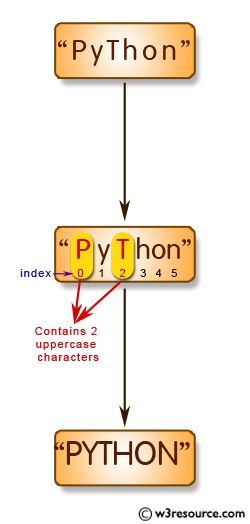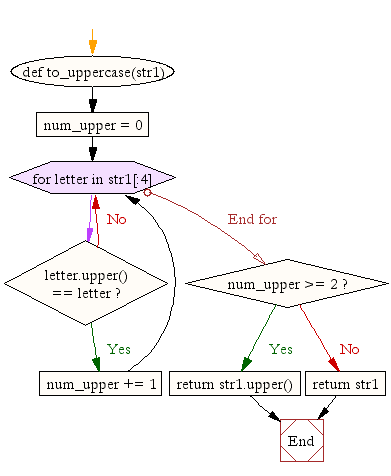﻿ Python: Convert a string to all uppercase if it contains at least 2 uppercase characters in the first 4 characters - w3resource# Python: Convert a string to all uppercase if it contains at least 2 uppercase characters in the first 4 characters

## Python String: Exercise-21 with Solution

Write a Python function to convert a given string to all uppercase if it contains at least 2 uppercase characters in the first 4 characters.Sample Solution:-

Python Code:

``````def to_uppercase(str1):
num_upper = 0
for letter in str1[:4]:
if letter.upper() == letter:
num_upper += 1
if num_upper >= 2:
return str1.upper()
return str1

print(to_uppercase('Python'))
print(to_uppercase('PyThon'))
```
```

Sample Output:

```Python
PYTHON
```

Flowchart:## Visualize Python code execution:

The following tool visualize what the computer is doing step-by-step as it executes the said program:

Python Code Editor:

Have another way to solve this solution? Contribute your code (and comments) through Disqus.

What is the difficulty level of this exercise?

Test your Python skills with w3resource's quiz

﻿

## Python: Tips of the Day

Unknown Arguments Using *arguments:

If your function can take in any number of arguments then add a * in front of the parameter name:

```def myfunc(*arguments):
for a in arguments:
print a
myfunc(a)
myfunc(a,b)
myfunc(a,b,c)
```